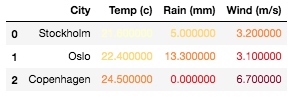Styler.text_gradient(cmap='PuBu', low=0, high=0, axis=0, subset=None, vmin=None, vmax=None, gmap=None)[source]

Color the text in a gradient style.

The text color is determined according to the data in each column, row or frame, or by a given gradient map. Requires matplotlib.

Parameters
cmapstr or colormap

Matplotlib colormap.

lowfloat

Compress the color range at the low end. This is a multiple of the data range to extend below the minimum; good values usually in [0, 1], defaults to 0.

highfloat

Compress the color range at the high end. This is a multiple of the data range to extend above the maximum; good values usually in [0, 1], defaults to 0.

axis{0 or ‘index’, 1 or ‘columns’, None}, default 0

Apply to each column (`axis=0` or `'index'`), to each row (`axis=1` or `'columns'`), or to the entire DataFrame at once with `axis=None`.

subsetlabel, array-like, IndexSlice, optional

A valid 2d input to DataFrame.loc[<subset>], or, in the case of a 1d input or single key, to DataFrame.loc[:, <subset>] where the columns are prioritised, to limit `data` to before applying the function.

text_color_thresholdfloat or int

This argument is ignored (only used in background_gradient). Luminance threshold for determining text color in [0, 1]. Facilitates text visibility across varying background colors. All text is dark if 0, and light if 1, defaults to 0.408.

vminfloat, optional

Minimum data value that corresponds to colormap minimum value. If not specified the minimum value of the data (or gmap) will be used.

New in version 1.0.0.

vmaxfloat, optional

Maximum data value that corresponds to colormap maximum value. If not specified the maximum value of the data (or gmap) will be used.

New in version 1.0.0.

gmaparray-like, optional

Gradient map for determining the text colors. If not supplied will use the underlying data from rows, columns or frame. If given as an ndarray or list-like must be an identical shape to the underlying data considering `axis` and `subset`. If given as DataFrame or Series must have same index and column labels considering `axis` and `subset`. If supplied, `vmin` and `vmax` should be given relative to this gradient map.

New in version 1.3.0.

Returns
selfStyler

`Styler.background_gradient`

Color the background in a gradient style.

Notes

When using `low` and `high` the range of the gradient, given by the data if `gmap` is not given or by `gmap`, is extended at the low end effectively by map.min - low * map.range and at the high end by map.max + high * map.range before the colors are normalized and determined.

If combining with `vmin` and `vmax` the map.min, map.max and map.range are replaced by values according to the values derived from `vmin` and `vmax`.

This method will preselect numeric columns and ignore non-numeric columns unless a `gmap` is supplied in which case no preselection occurs.

Examples

```>>> df = pd.DataFrame(columns=["City", "Temp (c)", "Rain (mm)", "Wind (m/s)"],
...                   data=[["Stockholm", 21.6, 5.0, 3.2],
...                         ["Oslo", 22.4, 13.3, 3.1],
...                         ["Copenhagen", 24.5, 0.0, 6.7]])
```

Shading the values column-wise, with `axis=0`, preselecting numeric columns

```>>> df.style.text_gradient(axis=0)
```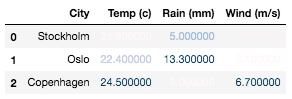Shading all values collectively using `axis=None`

```>>> df.style.text_gradient(axis=None)
```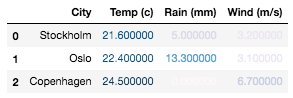Compress the color map from the both `low` and `high` ends

```>>> df.style.text_gradient(axis=None, low=0.75, high=1.0)
```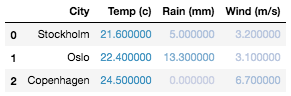Manually setting `vmin` and `vmax` gradient thresholds

```>>> df.style.text_gradient(axis=None, vmin=6.7, vmax=21.6)
```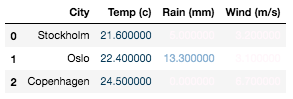Setting a `gmap` and applying to all columns with another `cmap`

```>>> df.style.text_gradient(axis=0, gmap=df['Temp (c)'], cmap='YlOrRd')
```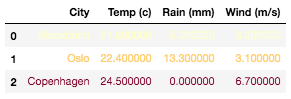Setting the gradient map for a dataframe (i.e. `axis=None`), we need to explicitly state `subset` to match the `gmap` shape

```>>> gmap = np.array([[1,2,3], [2,3,4], [3,4,5]])